CBSE Class 12 Sample Paper for 2022 Boards (MCQ Based - for Term 1)

Class 12
Solutions of Sample Papers and Past Year Papers - for Class 12 Boards

## (a) (-3√3 b)/a 2  (b) (-2√3 b)/a (c) (-3√3 b)/a  (d) (-b)/(3√3 a 2 )

This question is inspired from Question 31 (Choice 2) - CBSE Class 12 Sample Paper for 2021 Boards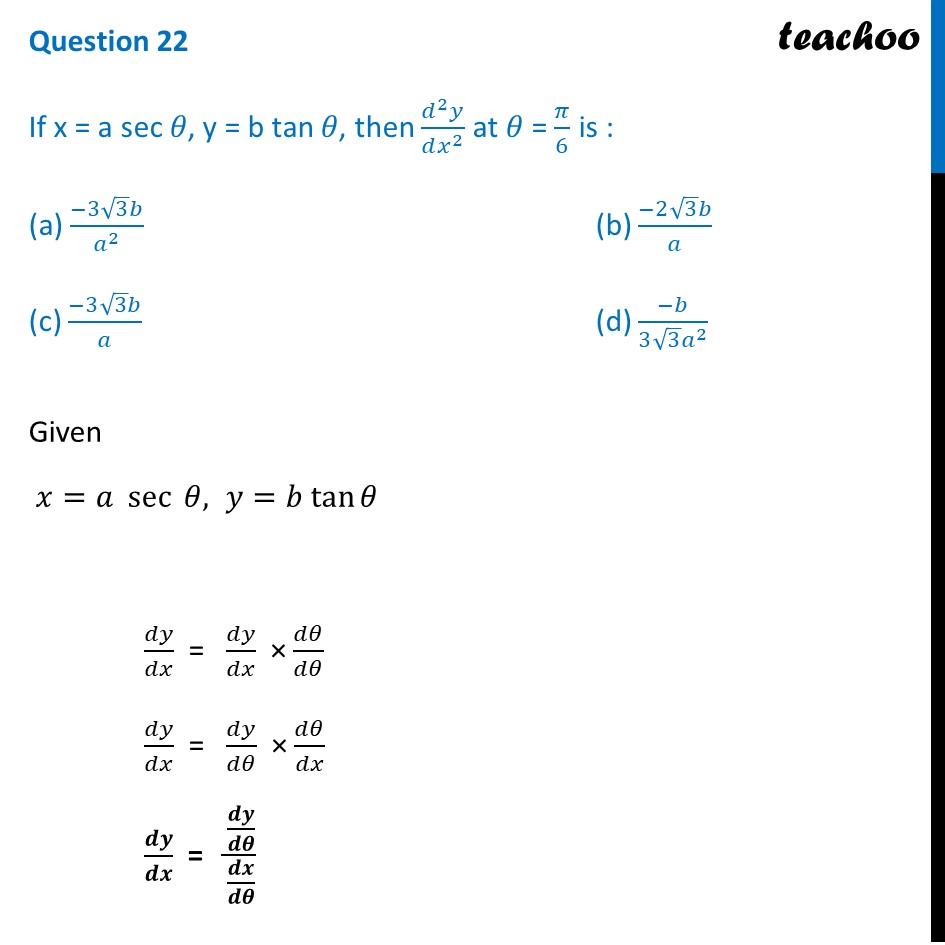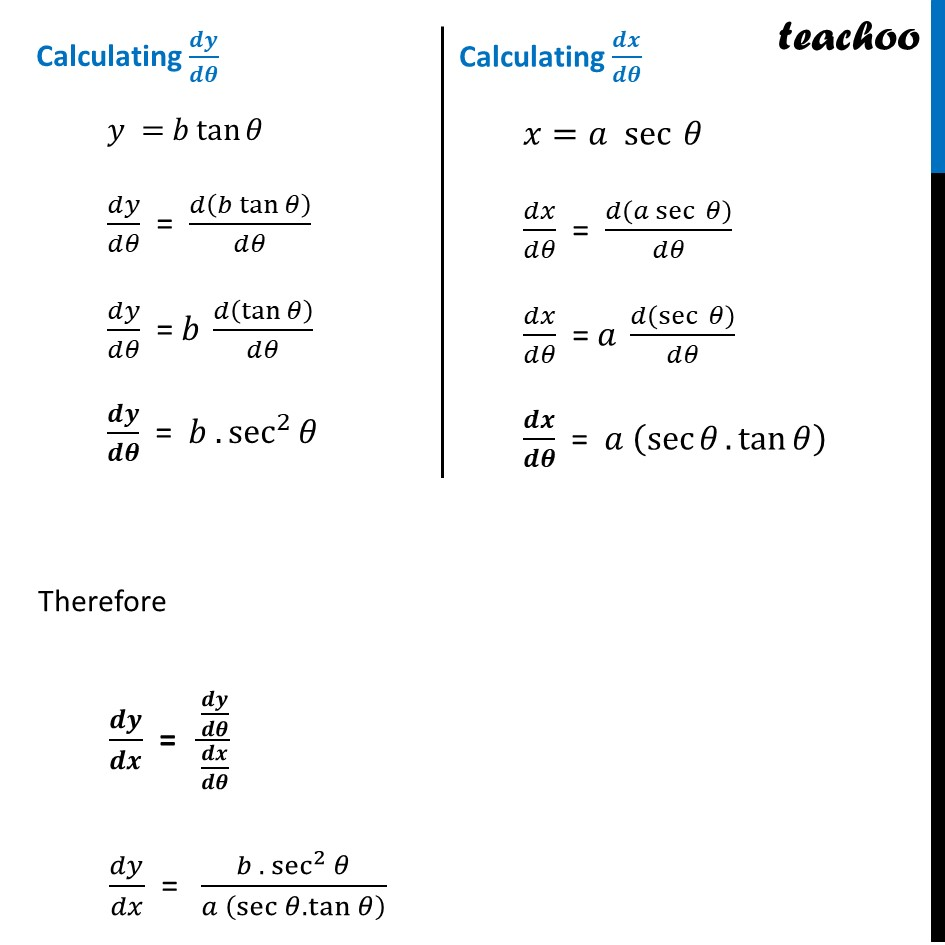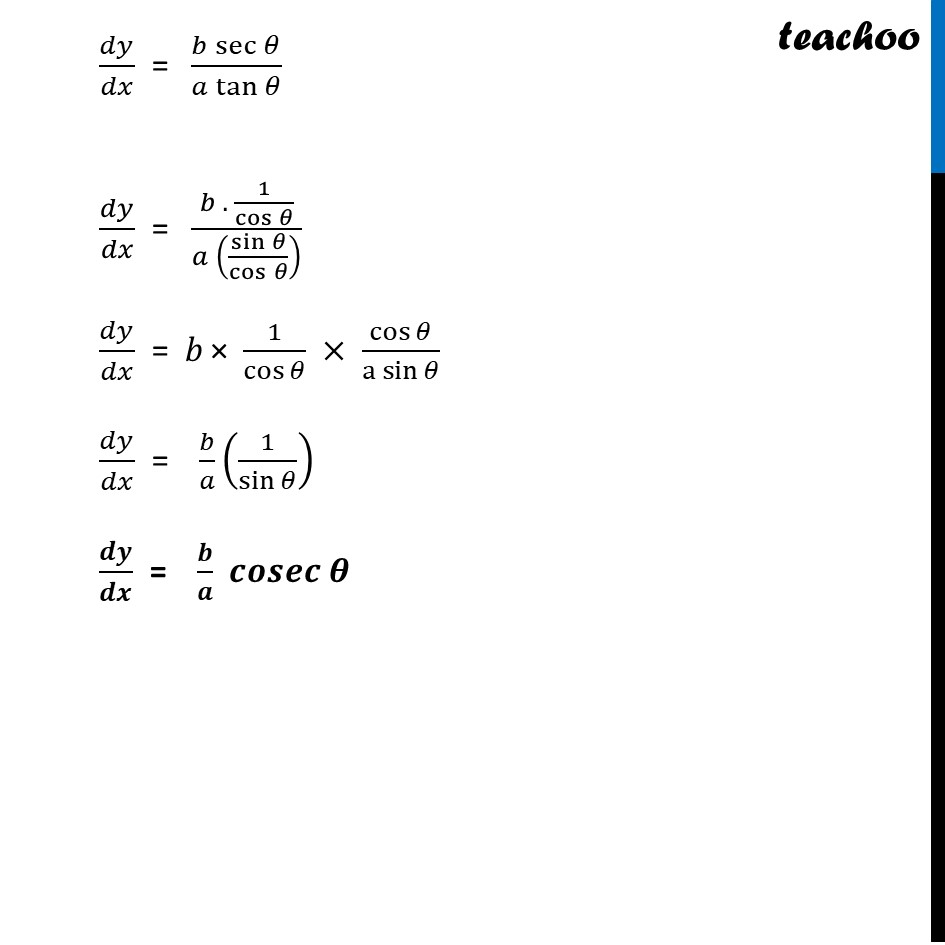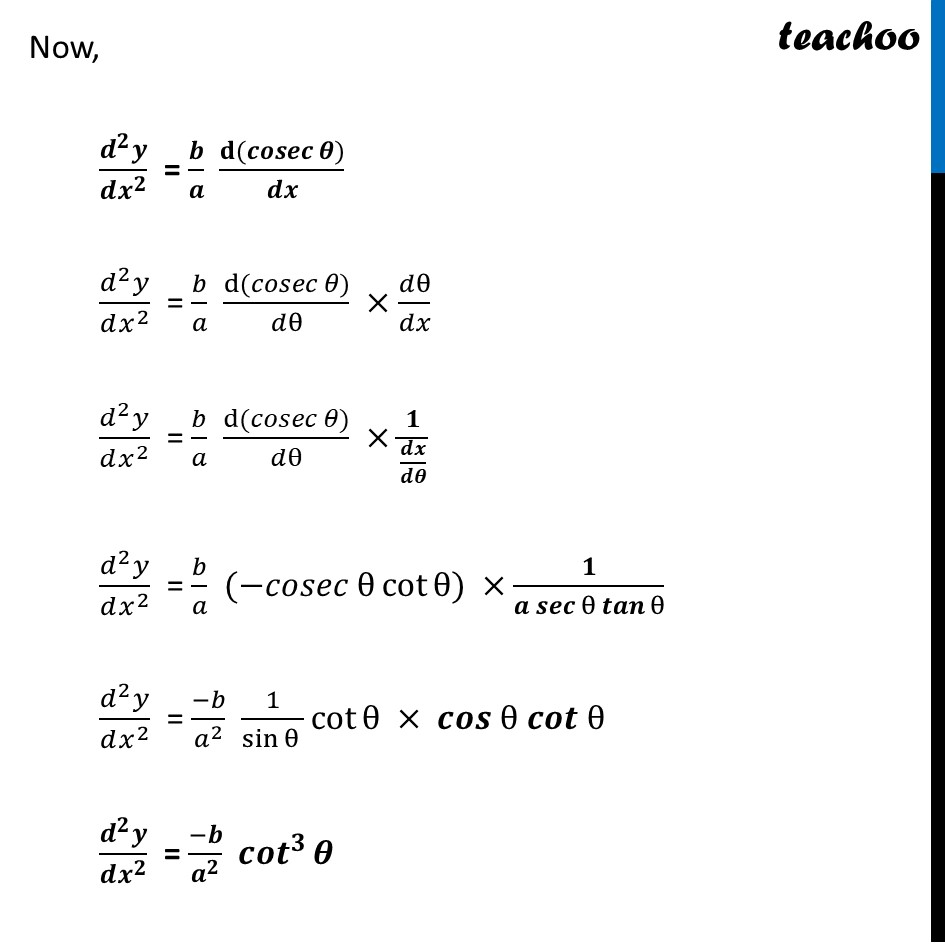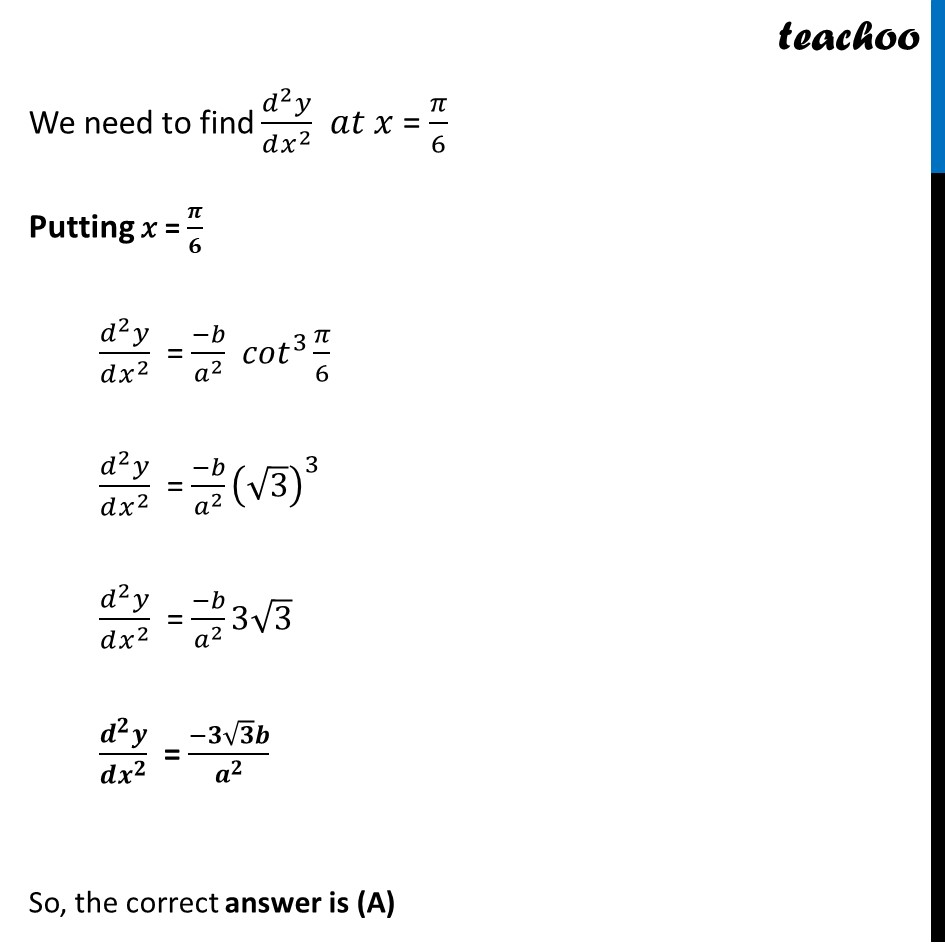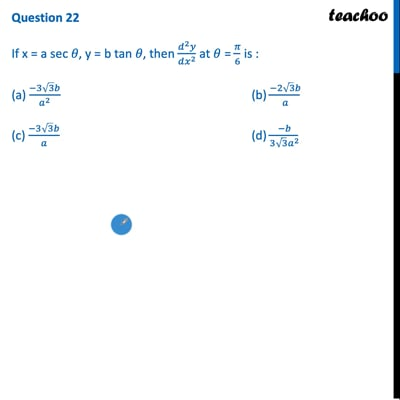This video is only available for Teachoo black users

Introducing your new favourite teacher - Teachoo Black, at only ₹83 per month

### Transcript

Question 22 If x = a sec 𝜃, y = b tan 𝜃, then (𝑑^2 𝑦)/(𝑑𝑥^2 ) at 𝜃 = 𝜋/6 is : (a) (−3√3 𝑏)/𝑎^2 (b) (−2√3 𝑏)/𝑎 (c) (−3√3 𝑏)/𝑎 (d) (−𝑏)/(3√3 𝑎^2 ) Given 𝑥=𝑎 sec ⁡𝜃, 𝑦=𝑏 tan⁡𝜃 𝑑𝑦/𝑑𝑥 = 𝑑𝑦/𝑑𝑥 × 𝑑𝜃/𝑑𝜃 𝑑𝑦/𝑑𝑥 = 𝑑𝑦/𝑑𝜃 × 𝑑𝜃/𝑑𝑥 𝒅𝒚/𝒅𝒙 = (𝒅𝒚/𝒅𝜽)/(𝒅𝒙/𝒅𝜽) Calculating 𝒅𝒚/𝒅𝜽 𝑦 = 𝑏 tan⁡𝜃 𝑑𝑦/𝑑𝜃 = 𝑑(𝑏 tan⁡𝜃 )/𝑑𝜃 𝑑𝑦/𝑑𝜃 = 𝑏 𝑑(tan⁡𝜃 )/𝑑𝜃 𝒅𝒚/𝒅𝜽 = 𝑏 .sec^2⁡𝜃 Calculating 𝒅𝒙/𝒅𝜽 𝑥=𝑎 sec ⁡𝜃 𝑑𝑥/𝑑𝜃 = 𝑑(𝑎 sec ⁡𝜃)/𝑑𝜃 𝑑𝑥/𝑑𝜃 = 𝑎 𝑑(sec ⁡𝜃)/𝑑𝜃 𝒅𝒙/𝒅𝜽 = 𝑎 (sec⁡𝜃.tan⁡𝜃 ) 𝑑𝑦/𝑑𝑥 = (𝑏 sec⁡𝜃)/(𝑎 tan⁡𝜃 ) 𝑑𝑦/𝑑𝑥 = (𝑏 . 1/cos⁡𝜃 )/(𝑎 (sin⁡𝜃/cos⁡𝜃 ) ) 𝑑𝑦/𝑑𝑥 = 𝑏 × 1/cos⁡𝜃 × cos⁡𝜃/〖a sin〗⁡𝜃 𝑑𝑦/𝑑𝑥 = 𝑏/𝑎 (1/sin⁡𝜃 ) 𝒅𝒚/𝒅𝒙 = 𝒃/𝒂 𝒄𝒐𝒔𝒆𝒄 𝜽 Now, (𝒅^𝟐 𝒚)/(𝒅𝒙^𝟐 ) = 𝒃/𝒂 (𝐝(𝒄𝒐𝒔𝒆𝒄 𝜽))/𝒅𝒙 (𝑑^2 𝑦)/(𝑑𝑥^2 ) = 𝑏/𝑎 (d(𝑐𝑜𝑠𝑒𝑐 𝜃))/𝑑θ ×𝑑θ/𝑑𝑥 (𝑑^2 𝑦)/(𝑑𝑥^2 ) = 𝑏/𝑎 (d(𝑐𝑜𝑠𝑒𝑐 𝜃))/𝑑θ ×𝟏/(𝒅𝒙/𝒅𝜽) (𝑑^2 𝑦)/(𝑑𝑥^2 ) = 𝑏/𝑎 (−𝑐𝑜𝑠𝑒𝑐 θ cot⁡θ) ×𝟏/(𝒂 𝒔𝒆𝒄 θ 𝒕𝒂𝒏 θ) (𝑑^2 𝑦)/(𝑑𝑥^2 ) = (−𝑏)/𝑎^2 1/sin⁡〖θ 〗 cot⁡θ × 𝒄𝒐𝒔 θ 𝒄𝒐𝒕 θ (𝒅^𝟐 𝒚)/(𝒅𝒙^𝟐 ) = (−𝒃)/𝒂^𝟐 〖𝒄𝒐𝒕〗^𝟑⁡𝜽 We need to find (𝑑^2 𝑦)/(𝑑𝑥^2 ) 𝑎𝑡 𝑥 = 𝜋/6 Putting 𝑥 = 𝝅/𝟔 (𝑑^2 𝑦)/(𝑑𝑥^2 ) = (−𝑏)/𝑎^2 〖𝑐𝑜𝑡〗^3⁡〖𝜋/6〗 (𝑑^2 𝑦)/(𝑑𝑥^2 ) = (−𝑏)/𝑎^2 (√3)^3 (𝑑^2 𝑦)/(𝑑𝑥^2 ) = (−𝑏)/𝑎^2 3√3 (𝒅^𝟐 𝒚)/(𝒅𝒙^𝟐 ) = (−𝟑√𝟑 𝒃)/𝒂^𝟐 So, the correct answer is (A)